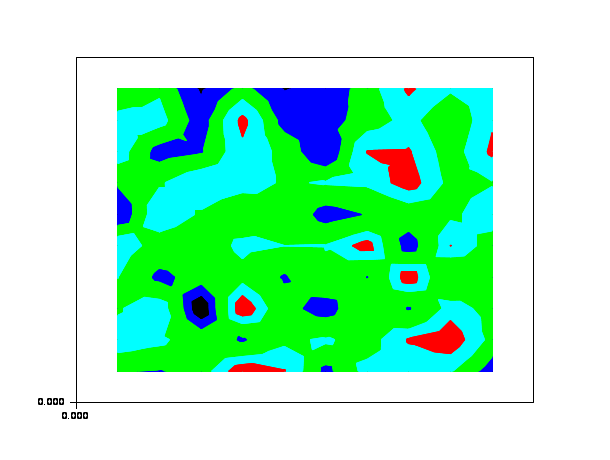Scilab Home page | Wiki | Bug tracker | Forge | Mailing list archives | ATOMS | File exchange
Change language to: Français - Português - 日本語 - Русский

See the recommended documentation of this function

Scilab Help >> Graphics > 2d_plot > contourf

# contourf

filled level curves of a surface on a 2D plot

### Calling Sequence

`contourf(x, y, z, nz, [style, strf, leg, rect, nax])`

### Arguments

x, y

two real row vectors of size `n1` and `n2`: the grid.

z

a real matrix of size `(n1,n2)`, the values of the function.

nz

the level values or the number of levels.

-

If `nz` is an integer, its value gives the number of level curves equally spaced from `zmin` to `zmax` as follows:

`z= zmin + (1:nz)*(zmax-zmin)/(nz+1)`Note: that the `zmin` and `zmax` levels are not drawn (generically they are reduced to points) but they can be added with

```[im,jm] = find(z == zmin);     // or zmax
plot2d(x(im)',y(jm)',-9,"000")```
-

If `nz` is a vector, `nz(i)` gives the value of the `i`-th level curve.

style, strf, leg, rect, nax

see `plot2d`. The argument `style` gives the colors which are to be used for level curves. It must have the same size as the number of levels.

### Description

`contourf` paints surface between two consecutive level curves of a surface `z=f(x,y)` on a 2D plot. The values of `f(x,y)` are given by the matrix `z` at the grid points defined by `x` and `y`.

You can change the format of the floating point number printed on the levels by using `xset("fpf",string)` where `string` gives the format in C format syntax (for example `string="%.3f"`). Use `string=""` to switch back to default format.

Enter the command `contourf()` to see a demo.

### Examples

`contourf(1:10,1:10,rand(10,10),5,1:5,"011"," ",[0,0,11,11])````function z=peaks(x, y)
x1=x(:).*.ones(1,size(y,'*'));
y1=y(:)'.*.ones(size(x,'*'),1);
z =  (3*(1-x1).^2).*exp(-(x1.^2) - (y1+1).^2) ...
- 10*(x1/5 - x1.^3 - y1.^5).*exp(-x1.^2-y1.^2) ...
- 1/3*exp(-(x1+1).^2 - y1.^2)
endfunction

function z=peakit()
x=-4:0.1:4;y=x;z=peaks(x,y);
endfunction

z=peakit();

levels=[-6:-1,-logspace(-5,0,10),logspace(-5,0,10),1:8];
m=size(levels,'*');
n = fix(3/8*m);
r = [(1:n)'/n; ones(m-n,1)];
g = [zeros(n,1); (1:n)'/n; ones(m-2*n,1)];
b = [zeros(2*n,1); (1:m-2*n)'/(m-2*n)];
h = [r g b];
xset('colormap',h);
xset('fpf',' ');
clf();
contourf([],[],z,[-6:-1,-logspace(-5,0,10),logspace(-5,0,10),1:8],0*ones(1,m))

xset('fpf','');
clf();
contourf([],[],z,[-6:-1,-logspace(-5,0,10),logspace(-5,0,10),1:8]);```• contour — level curves on a 3D surface
• contour2d — level curves of a surface on a 2D plot
• contour2di — compute level curves of a surface on a 2D plot
• plot2d — 2D plot